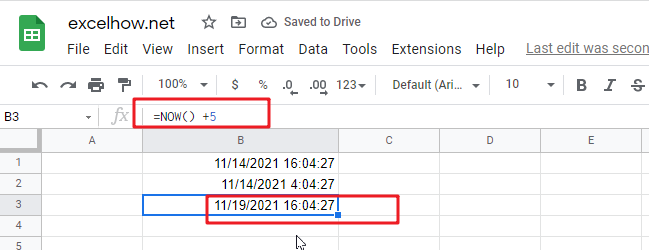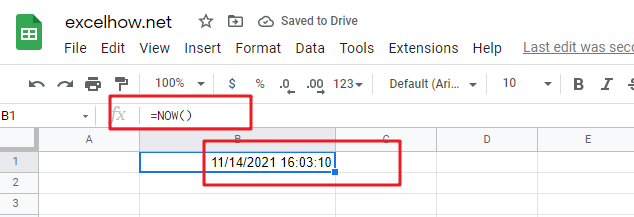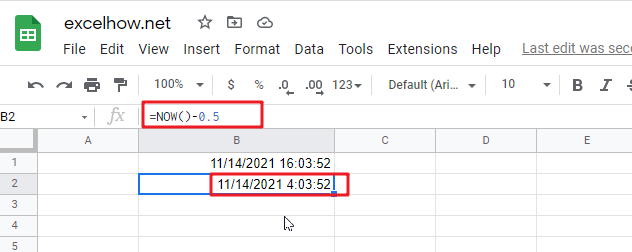This post will guide you how to use Google Sheets NOW function with syntax and examples.

## Description

The Google Sheets NOW function returns the serial number of the current date and time. And it will be updated each time when your worksheet is changed or opened. This function does not need to add any arguments.

The NOW function can be used to get the current date and time in google sheets. The purpose of this function is to get the current date and time and its returned values is a number that representing the date and time in google sheets.

The NOW function is a build-in function in Google Sheets and it is categorized as a Date function.

## Syntax

The syntax of the NOW function is as below:

=NOW ()

Note:

• A serial date is how the google sheets stores dates and it represents the number of days since 1900-01-01, so the January 1, 1900 date is serial number 1 by default.
• Numbers to the right of the decimal point in the serial number represent the time; numbers to the left represent the date. For example, the serial number 0.5 represents the time 12:00 noon. And the serial number 0.25 represents the time 6:00 AM.
•  If the NOW function does not update cell values when you expect it to, you might need to change settings that control when the sheet recalculates.
• Now function will be updated on every edit made to the spreadsheet and it will impact spreadsheet performance.
• NOW function will provide the current date and time. If you wish to create a date without the current time, using TODAY function.
• NOW function will always get the current date and time and the last time in the spreadsheet was recalculated, rather than remaining at the date and time when it was first entered.
• The date or time component of NOW function can be hidden by changing the number formatting.

## Google Sheets NOW Function Examples

The below examples will show you how to use google sheets NOW Function to return the current date and time.

1# get the current date and time, enter the following formula in Cell B1.

`=NOW()`2# get the date and time 12 hours ago, you can use the following formula:

`=NOW()-0.5`3# get the date and time 5 days in the future, just use the following formula:

`=NOW() + 5`